IMO Shortlist 2008 problem G2

Kvaliteta:
Avg: 0,0
Težina:
Avg: 6,0
Given trapezoid$ABCD$ with parallel sides$AB$ and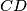$CD$, assume that there exist points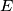$E$ on line$BC$ outside segment$BC$, and$F$ inside segment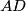$AD$ such that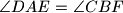$\angle DAE = \angle CBF$. Denote by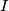$I$ the point of intersection of$CD$ and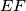$EF$, and by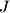$J$ the point of intersection of$AB$ and$EF$. Let$K$ be the midpoint of segment$EF$, assume it does not lie on line$AB$. Prove that$I$ belongs to the circumcircle of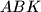$ABK$ if and only if$K$ belongs to the circumcircle of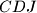$CDJ$.

Proposed by Charles Leytem, Luxembourg
Izvor: Međunarodna matematička olimpijada, shortlist 2008